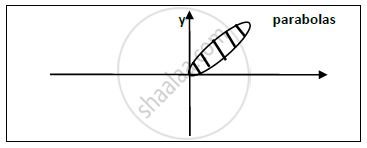# Find the Volume Enclosed by the Cylinder Y 2 = X and Y = X 2 Cut off by the Planes Z = 0, X+Y+Z=2. - Applied Mathematics 2

Sum

Find the volume enclosed by the cylinder y^2=x and y=x^2 Cut off by the planes z = 0, x+y+z=2.

#### Solution

The solid is bounded by the parabolas y^2=x, y=x^2 in the x y plane.In x-y-z plane x+y+z =2 is top base.
The volume between this curves is given by ,
V = ∫∫𝒛 𝒅𝒙 𝒅𝒚= ∫∫(𝟐−𝒙−𝒚)𝒅𝒙𝒅𝒚
From the diagram we can conclude that the intersection point of both Parabolas are (0,0),(1,1).

therefore "V"=int_0^1int_(x^2)^sqrtx(2-x-y)dxdy

=int_0^1(2y-xy-y^2/2)_(x^2)^sqrtxdx

=int_0^1[(2sqrtx-xsqrtx-x/2)-(2x^2-x^3-x^4/2)]dx

=[(4x^(3/2))/3-(2x^(5/2))/5-x^2/4-(2x^3)/3+x^4/4+x^5/10]_0^1

therefore "V"=11/30

Concept: Triple Integration Definition and Evaluation
Is there an error in this question or solution?